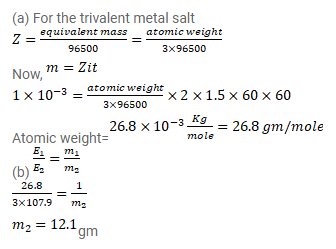# Two voltameters, one having a solution of silver salt and

Question:

Two voltameters, one having a solution of silver salt and the other of a trivalent-metal salt, are connected in series and a current of $2 \mathrm{~A}$ is maintained for $1.50$ hours. It is found that $1.00 \mathrm{~g}$ of the trivalent-metal is deposited.

(a) What is the atomic weight of the trivalent metal?

(b) How much silver is deposited during this period? Atomic weight of silver is $107.9 \mathrm{~g} / \mathrm{mole}$.

Solution: